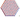## 六阶模幻方

Tue, 14th January 2020Edit on Githubmodular

# 摘要

### $Z_6^2$上的最优幻方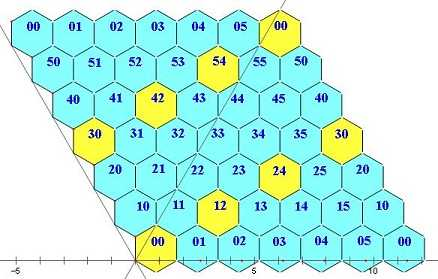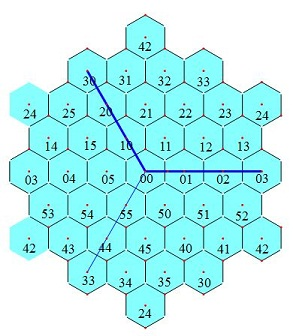$f(x,y)+f(x,y+1)+f(x,y+2)+...+f(x,y+5)=6A$($A$是36个填入数的平均值）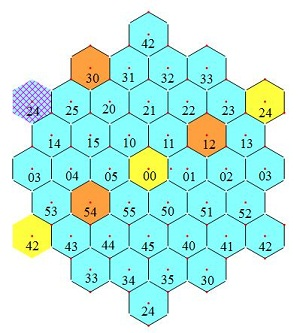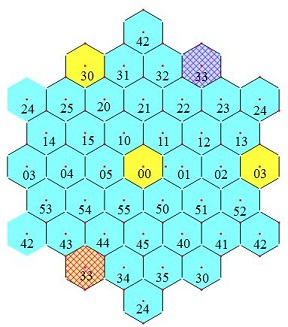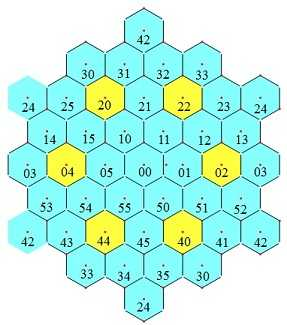# 更多规律分析

hujunhua接着给出了更多的规律分析 :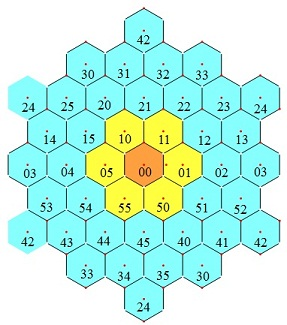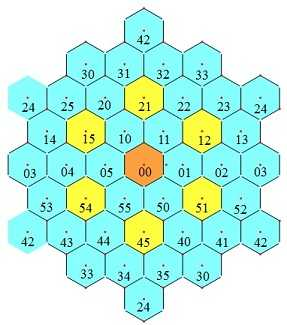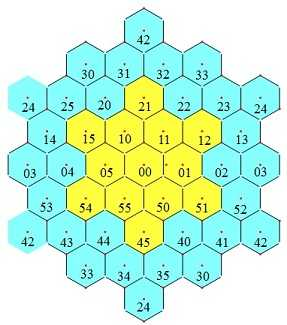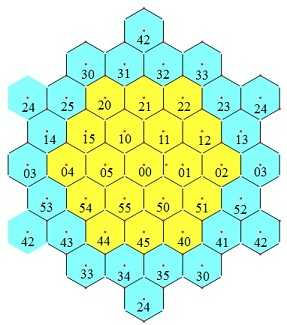# 参数方案

6阶零和模幻方的定义中给出的36个约束方程的秩为24，所以参数解的维数为12. 上图中我们选两个白六角形的六个角a1,b1,...,f1, a2,b2, ..., f2为参数，其它格就可以由上述导出律得到。s1=-(a1+b1+...+f1)/2, s2=-(a2+b2+...+f2)/2, s3=-(s1+s2), a3=-(a1+a2),....

mathe后来也给出了类似的分析结果

# 单孔6阶模幻方不存在

hujunhua先开始用程序进行搜索 ，发现52小时才运行了大概5%的进度，没有找到任何合法的结果，终止了代码的运行。
mathe开始用C编写代码穷举，但是代码里面出现多次bug, 屡败屡战，终于穷举证明了只有一个空缺的6阶模幻方是不存在的

# 最小6阶模幻方

0~39: Find:

a_(0,0)=28 a_(0,1)=7 a_(0,2)=38 a_(0,3)=8 a_(0,4)=29 a_(0,5)=10
a_(1,0)=17 a_(1,1)=25 a_(1,2)=13 a_(1,3)=22 a_(1,4)=19 a_(1,5)=24
a_(2,0)=21 a_(2,1)=18 a_(2,2)=27 a_(2,3)=15 a_(2,4)=23 a_(2,5)=16
a_(3,0)=11 a_(3,1)=32 a_(3,2)=2 a_(3,3)=33 a_(3,4)=12 a_(3,5)=30
a_(4,0)=4 a_(4,1)=35 a_(4,2)=9 a_(4,3)=37 a_(4,4)=1 a_(4,5)=34
a_(5,0)=39 a_(5,1)=3 a_(5,2)=31 a_(5,3)=5 a_(5,4)=36 a_(5,5)=6

Find:

a_(0,0)=4 a_(0,1)=3 a_(0,2)=38 a_(0,3)=22 a_(0,4)=23 a_(0,5)=30
a_(1,0)=11 a_(1,1)=35 a_(1,2)=31 a_(1,3)=8 a_(1,4)=19 a_(1,5)=16
a_(2,0)=21 a_(2,1)=32 a_(2,2)=9 a_(2,3)=5 a_(2,4)=29 a_(2,5)=24
a_(3,0)=17 a_(3,1)=18 a_(3,2)=2 a_(3,3)=37 a_(3,4)=36 a_(3,5)=10
a_(4,0)=28 a_(4,1)=25 a_(4,2)=27 a_(4,3)=33 a_(4,4)=1 a_(4,5)=6
a_(5,0)=39 a_(5,1)=7 a_(5,2)=13 a_(5,3)=15 a_(5,4)=12 a_(5,5)=34

hujunhua将它们转化为图案后发现它们其实是等价的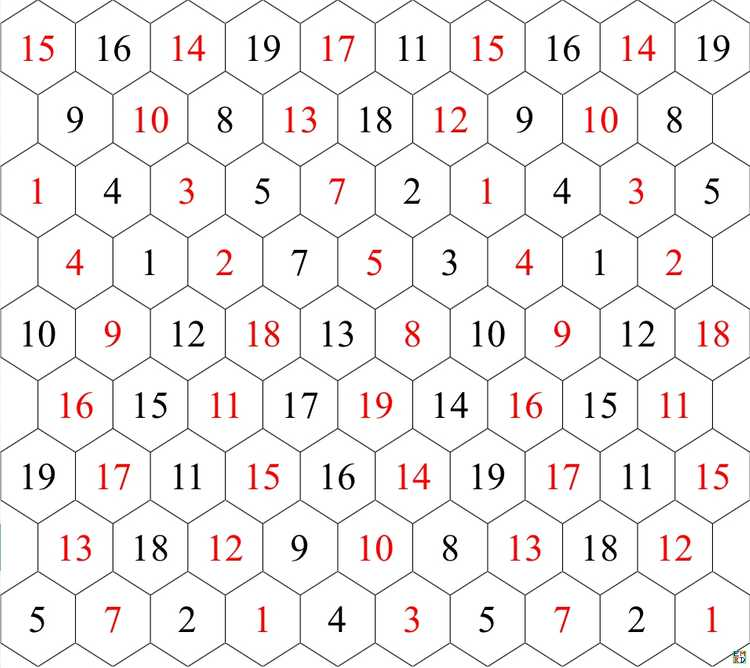mathe还验证 了也不存在双孔6阶模幻方，并且穷举出所有可能的三孔6阶模幻方

# 美图秀

hujunhua首先对数据进行了分析处理，得出 :

{0~38}的48个，化为{-19~19}后全都为非对称数组（即都不含0洞）。
{洞组, 频数｝如下： {{-18, -12, -6}, 24}，
{{-16, -14, -6}, 12}
{{-14, -12, -10},12}。

{1~39}的6792个，化为{-19~19}后，全部为零和幻方，其中6624个为对称数组，其余168个为非对称数组（含0）。
{洞组, 频数｝
{{-2, 0, 2}, 180},
{{-4, 0, 4}, 240},
{{-6, 0, 6}, 972},
{{-8, 0, 8}, 540},
{{-10, 0, 10}, 768},
{{-12, 0, 12}, 888},
{{-14, 0, 14},312},
{{-16, 0, 16}, 780},
{{-18, 0, 18}, 1944},(\times 这个洞组的最多，先前104例中居然没碰到\times )。
{{-10, -8, 18}, 36},（\times 非对称洞组\times ）
{{-18, 8, 10}, 36},(\times 与上行对称，重合情况有待分析\times ）
{{-10, -6, 16}, 12},
{{-16, 6, 10}, 12}, (\times 与上行对称，重合情况有待分析\times ）
{{-14, -4, 18},36},
{{-18, 4, 14}, 36}}(\times 与上行对称，重合情况有待分析\times ）

{{-19, -13, -7}, 2}
{{-17, -15, -7}, 1}
{{-15, -13, -11}, 1}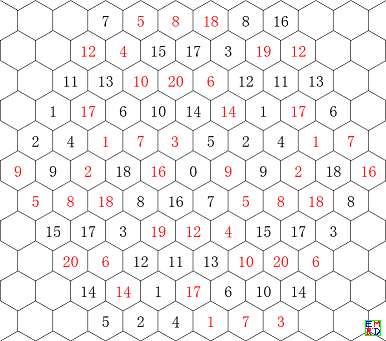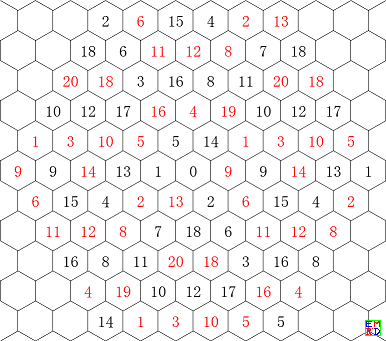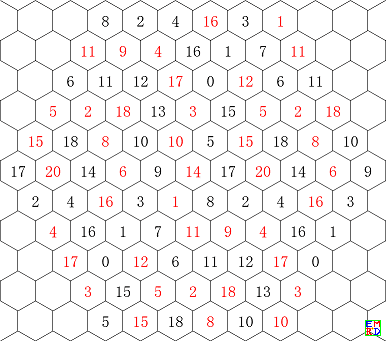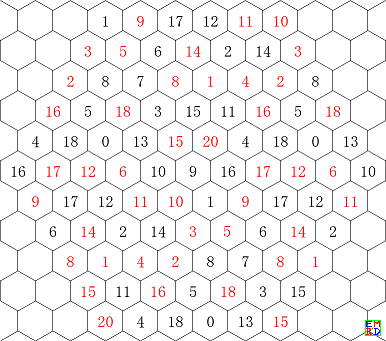RulesArray =Solve[x + y + z == 15 && 0 <= x <= 10 && 0 <= y <= 10 && 0 <= z <= 10, {x, y, z}, Integers];
mf[n_] := m13906[[n, Mod[5 - z, 6, 1], Mod[y + 1, 6, 1]]]
Graphics3D[{Sphere[{x, y, z}], Text[Style[Abs@mf, Medium, Bold, If[mf > 0, Black, Red]], {x, y, z}]} /. RulesArray, ViewPoint -> {100, 100, 100}, Boxed -> False]

{{-10, -8, 18}, 36}
{{-18, 8, 10}, 36}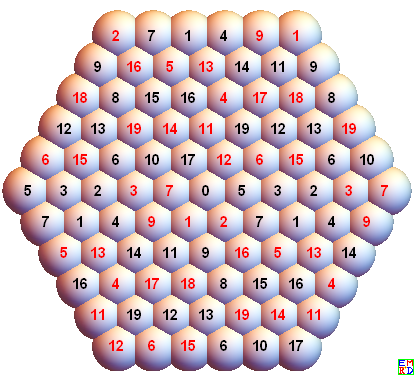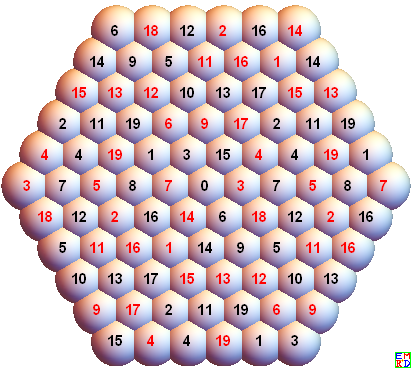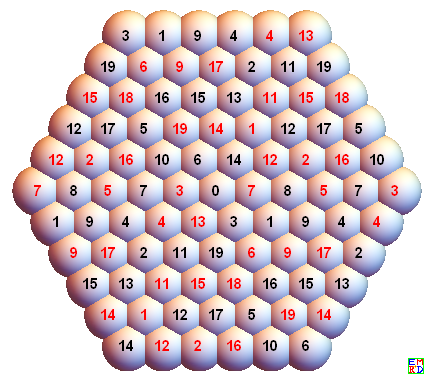{{-10, -6, 16}, 12},
{{-16, 6, 10}, 12}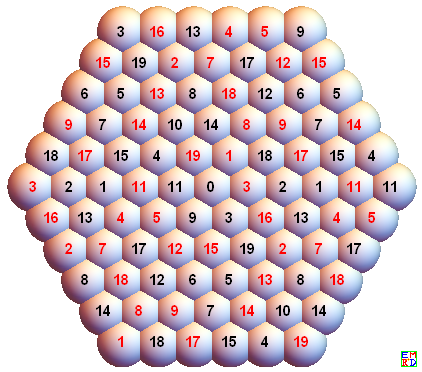{{-14, -4, 18},36},
{{-18, 4, 14}, 36}}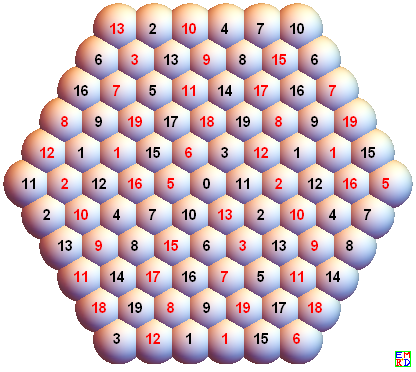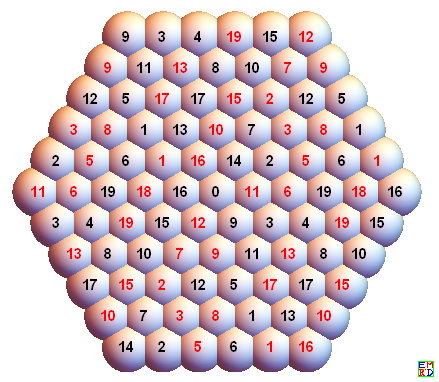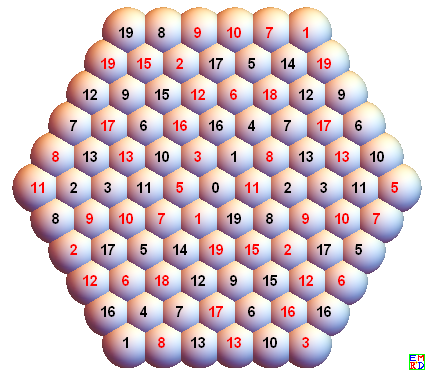{洞组, 频数｝
{{-2, 0, 2}, 180},
{{-4, 0, 4}, 240},
{{-6, 0, 6}, 972},
{{-8, 0, 8}, 540},
{{-10, 0, 10}, 768},
{{-12, 0, 12}, 888},
{{-14, 0, 14}, 312},
{{-16, 0, 16}, 780},
{{-18, 0, 18}, 1944}

### {{-2, 0, 2}, 180}去重:

mathe是固定 a(3, 2)=2，化为对称数组后即红18不动。下面画图时，a(3, 2)位于中心。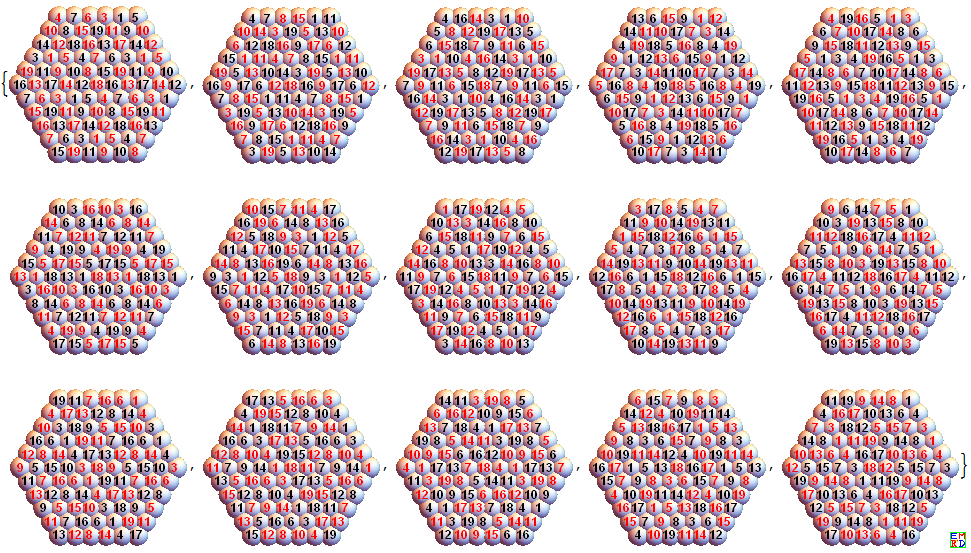### {{-4, 0, 4}, 240}去重，16个本原解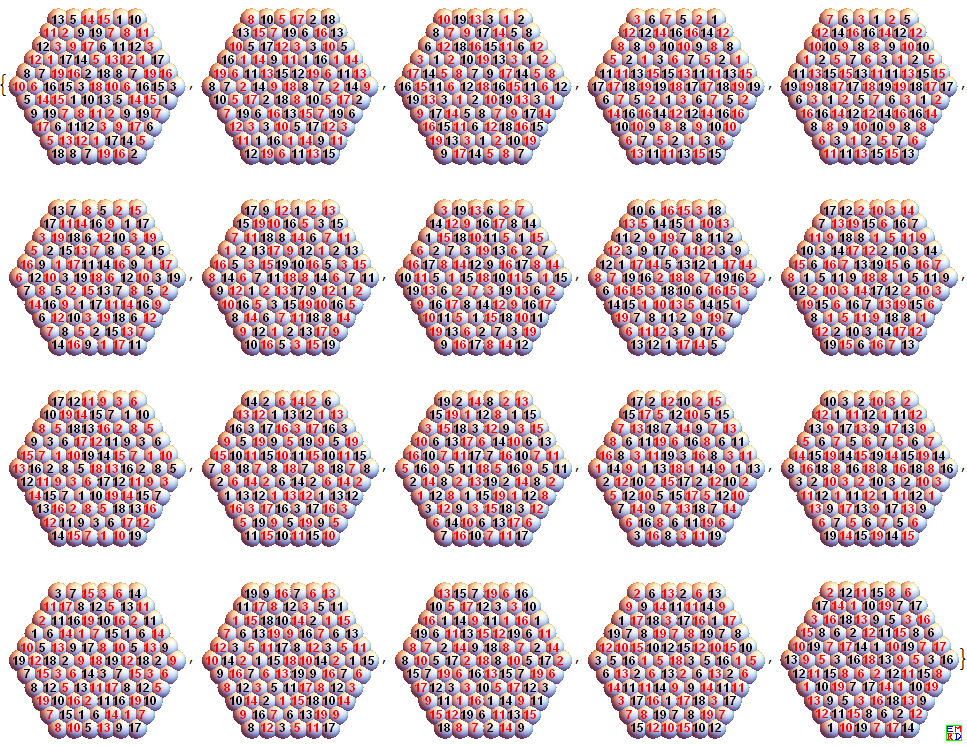1 与 9，2 与 18， 4 与 13， 5 与14 是取反重复的。
4与13， 5与14 的相反数对关系很诡异，即我前面所称的分块对称，其实这四个貌似也有某种取反自对称。

### 中心对称12图

mathe 给的3孔数组幻方中，第1个就是中心对称幻方（见27楼和34楼），所以最初以为会有很多中心对称幻方的。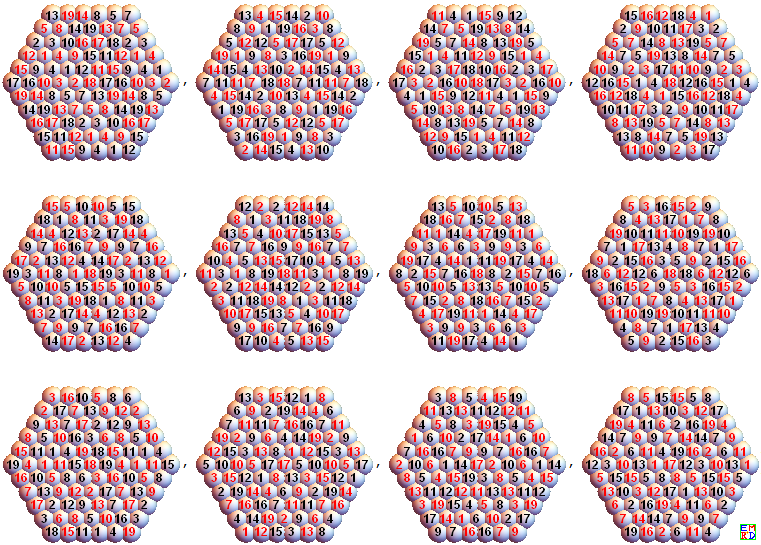# 去重汇总

{-2,0,2} 180 15 15 0 0 15
{-4,0,4} 240 20 10 0 5对 15
{-6,0,6} 972 81 37 6 19对 62
{-8,0,8} 540 45 7 0 19对 26
{-10,0,10} 768 64 14 0 25对 39
{-12,0,12} 888 74 49 1 12对 62
{-14,0,14} 312 26 20 2 2对 24
{-16,0,16} 780 65 29 0 18对 47
{-18,0,18} 1944 162 87 3 36对 126

## 不对称的种种（一）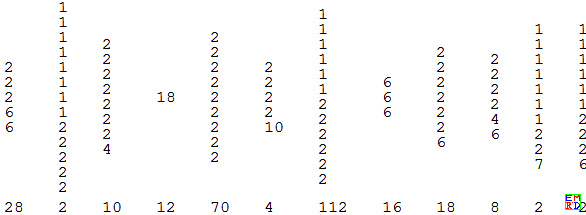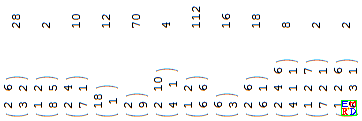中点权数表的合并横放版

## 不对称的种种（二）

#是幻方 x 中的一个数，相反数偶的向量= Position(x, #)-Position(x, -#)(mod 6, -2)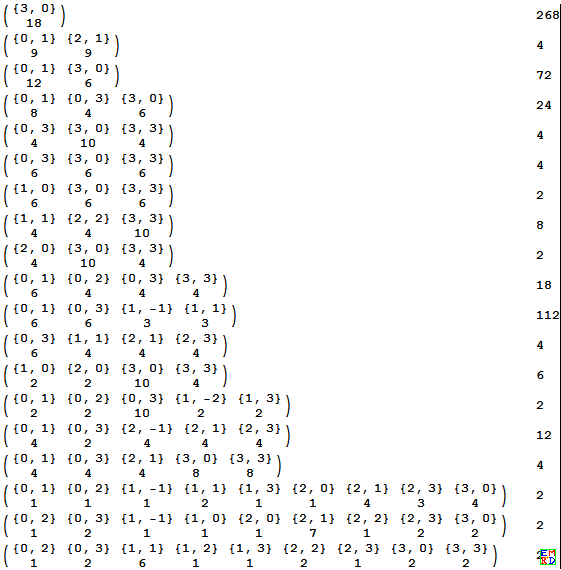## 不对称的种种（三）

1、平移对称
2、中心对称
3、频数为112者，说明这种不再细分了。

Github